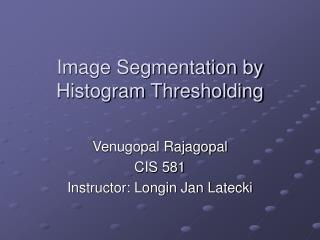DownloadDownload PresentationImage Segmentation by Histogram Thresholding

# Image Segmentation by Histogram Thresholding

Download Presentation## Image Segmentation by Histogram Thresholding

- - - - - - - - - - - - - - - - - - - - - - - - - - - E N D - - - - - - - - - - - - - - - - - - - - - - - - - - -
##### Presentation Transcript

1. Image Segmentation by Histogram Thresholding Venugopal Rajagopal CIS 581 Instructor: Longin Jan Latecki

2. Image Segmentation • Segmentation divides an image into its constituent regions or objects. • Segmentation of non trivial images is one of the difficult task in image processing. Still under research. • Segmentation accuracy determines the eventual success or failure of computerized analysis procedure. • Example Application: Automated inspection of electronic assemblies. (mother boards)

3. Segmentation Algorithms • Segmentation algorithms are based on one of two basic properties of intensity values discontinuity and similarity. • First category is to partition an image based on abrupt changes in intensity, such as edges in an image. • Second category are based on partitioning an image into regions that are similar according to a predefined criteria. Histogram Thresholding approach falls under this category.

4. Histograms • Histogram are constructed by splitting the range of the data into equal-sized bins (called classes). Then for each bin, the number of points from the data set that fall into each bin are counted. • Vertical axis: Frequency (i.e., counts for each bin) • Horizontal axis: Response variable • In image histograms the pixels form the horizontal axis • In Matlab histograms for images can be constructed using the imhist command.

5. Thresholding - Foundation • Suppose that the gray-level histogram corresponds to an image, f(x,y), composed of dark objects in a light background, in such a way that object and background pixels have gray levels grouped into two dominant modes. One obvious way to extract the objects from the background is to select a threshold ‘T’ that separates these modes. Then any point (x,y) for which f(x,y) > T is called an object point, otherwise, the point is called a background point.

6. Example

7. Foundation (contd.) • If two dominant modes characterize the image histogram, it is called a bimodal histogram. Only one threshold is enough for partitioning the image. • If for example an image is composed of two types of light objects on a dark background, three or more dominant modes characterize the image histogram.

8. Foundation (contd.) • In such a case the histogram has to be partitioned by multiple thresholds. • Multilevel thresholding classifies a point (x,y) as belonging to one object class if T1 < (x,y) <= T2, to the other object class if f(x,y) > T2 and to the background if f(x,y) <= T1.

9. Thresholding • Basic Global Thresholding: 1)Select an initial estimate for T 2)Segment the image using T. This will produce two groups of pixels. G1 consisting of all pixels with gray level values >T and G2 consisting of pixels with values <=T. 3)Compute the average gray level values mean1 and mean2 for the pixels in regions G1 and G2. 4)Compute a new threshold value T=(1/2)(mean1 +mean2) 5)Repeat steps 2 through 4 until difference in T in successive iterations is smaller than a predefined parameter T0. • Basic Adaptive Thresholding: Images having uneven illumination makes it difficult to segment using histogram, this approach is to divide the original image into sub images and use the above said thresholding process to each of the sub images.

10. Thresholding (contd.) • In this project we have used a different method based on “Discrete Curve Evolution” to find thresholds in the histogram.

11. Thresholding – Colour Images • In colour images each pixel is characterized by three RGB values. • Here we construct a 3D histogram, and the basic procedure is analogous to the method used for one variable. • Histograms plotted for each of the colour values and threshold points are found.

12. Displaying objects in the Segmented Image • The objects can be distinguished by assigning a arbitrary pixel value or average pixel value to the regions separated by thresholds.

13. Experiments • Type of images used: 1) Two Gray scale image having bimodal histogram structure. 2) Gray scale image having multi-modal histogram structure. 3) Colour image having bimodal histogram structure. 4) Colour image having multi-modal histogram structure.

14. Gray Scale Image - bimodal Image of a Finger Print with light background

15. Bimodal - Histogram Image Histogram of finger print

16. Segmented Image Image after Segmentation

17. Gray Scale Image (2) - bimodal Image of rice with black background

18. Histogram Image histogram of rice

19. Segmented Image Image after segmentation

20. Gray Scale Image - Multimodal Original Image of lena

21. Multimodal Histogram Histogram of lena

22. Segmented Image Image after segmentation – we get a outline of her face, hat, shadow etc

23. Colour Image - bimodal Colour Image having a bimodal histogram

24. Histogram Histograms for the three colour spaces

25. Segmented Image Segmented image – giving us the outline of her face, hand etc

26. Colour Image - Multimodal Colour Image having multi-modal histogram

27. Histogram Image Histogram for the three colour spaces

28. Segmented Image

29. Experiments (contd.) Original Image, objective is to extract a line

30. Histogram Histogram giving us the six thresholds points, by plotting rows

31. Segmented Image

32. Segmented Image (contd.)

33. Conclusion • After segmenting the image, the objects can be extracted using edge detection techniques. • Image segmentation techniques are extensively used in Similarity Searches. (IDB) Thank You